# Model Test Paper - 2

## 30 Questions MCQ Test Science Olympiad Class 6 | Model Test Paper - 2

Description
This mock test of Model Test Paper - 2 for Class 6 helps you for every Class 6 entrance exam. This contains 30 Multiple Choice Questions for Class 6 Model Test Paper - 2 (mcq) to study with solutions a complete question bank. The solved questions answers in this Model Test Paper - 2 quiz give you a good mix of easy questions and tough questions. Class 6 students definitely take this Model Test Paper - 2 exercise for a better result in the exam. You can find other Model Test Paper - 2 extra questions, long questions & short questions for Class 6 on EduRev as well by searching above.
QUESTION: 1

Solution:
QUESTION: 2

Solution:
QUESTION: 3

### Which of these is a correct statement?

Solution:
QUESTION: 4

Identify X and Y in the given Venn diagram and select the correct option.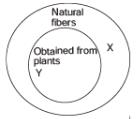Solution:
QUESTION: 5

Decantation process is used to separate:

Solution:
QUESTION: 6

Crude oil is purified using fractional distillation process. This method is based on:

Solution:
QUESTION: 7

Winnowing is used only for:

Solution:
QUESTION: 8

When the temperature of the solid is increased, the  energy of the particles increases.

Solution:
QUESTION: 9

Study this Venn diagram.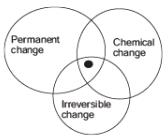The point at the center represents:

Solution:
QUESTION: 10

What is one common property among the following phenomena?
1. the rotation of blades of a fan
2. the blinking of traffic lights
3. the swinging of a pendulum

Solution:
QUESTION: 11

Shiva covered one of the leaves of his green plant shown below with a piece of black pastel paper. He then kept the plant in a dark place for 2 days.
After 2 days of darkness, he kept the plant in a bright sunny place for a day. At the end of the day, Shiva conducted an iodine test on the leaf.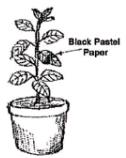Q. Which one of the diagrams below shows the result of the test?

Solution:
QUESTION: 12

Mary made the following statements about photosynthesis.Which of the statements is/are INCORRECT?

Solution:
QUESTION: 13

Plants excrete gases through:

Solution:
QUESTION: 14

The smallest time measured by a wrist watch accurately is:

Solution:
QUESTION: 15

When a drill bores a hole in a piece of wood, it demonstrates:

Solution:
QUESTION: 16

Two identical metal balls A and B moving in opposite directions hit each other at points X as shown in the figure. Changes are most likely to appear in their:

Solution:
QUESTION: 17

Adam shone a beam of light from a torch at two objects, X and Y, as shown in the diagram below.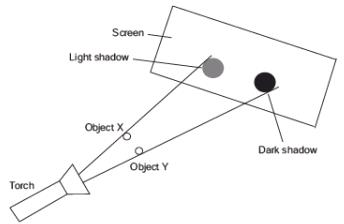The shadow formed by object X was much lighter than the one formed by object Y.

Q. Which of the following materials are X and Y likely to be made of?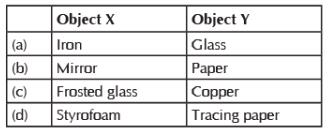Solution:
QUESTION: 18

A plane mirror reflects a pencil of light to form a real image. Then the pencil of light incident on the mirror is:

Solution:
QUESTION: 19

Kara performed an experiment. She positioned a tennis ball between a screen and a torch as shown below.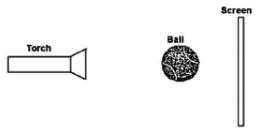She wrote down the steps she followed:
1. Switch on the torch.
2. Measure the height of the shadow of the tennis ball formed on the screen.
3. Move the tennis ball 5 cm closer to the torch.
4. Measure the height of the shadow again.
5. Repeat steps (3) and (4) twice, moving the tennis ball 5 cm closer to the torch each time.

Q. Which of the following states the correct hypothesis for the above experiment?

Solution:
QUESTION: 20

Sameer’s torch does not light up. This could be because of the following reason:

Solution:
QUESTION: 21

Bulb will glow in: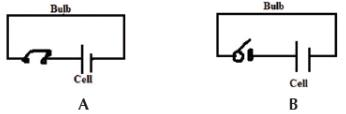Solution:
QUESTION: 22

In which of the following a permanent magnet not used?

Solution:
QUESTION: 23

The diagram below shows what happens when two objects, X and Y, are placed very close to each other on a piece of paper laced with iron fillings.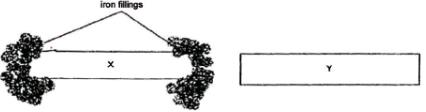Q. Which one of the following statement(s) is/are definitely true?
1. Object X is a magnet
2. Object X is made of copper
3. Object Y is made of non-magnetic material
4. Object Y is made of magnetic material

Solution:
QUESTION: 24

Which of the following groups of items is needed to make an electromagnet?

Solution:
QUESTION: 25

The needle of the compass is made of a magnet because it .

Solution:
QUESTION: 26

Four nails of different materials – iron, steel, copper, aluminum – were stroked with a magnet. Which of them will turn into a magnet?

Solution:
QUESTION: 27

Set-ups A and B below are placed on a table in the science room.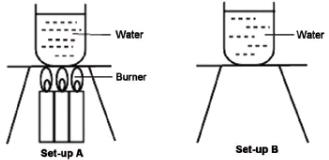Q. What are the similarities that can be observed in both set-ups after 45 minutes?

Solution:
QUESTION: 28

Which of the following situations show(s) that heat is lost?
1. When ice melts
2. When water vapour condenses
3. When water changes to ice
4. When a steel pot is left in the fridge

Solution:
QUESTION: 29

Early in the morning, Ryan observed that there were water droplets on the outside of cars, even though it did not rain the night before.

Q. What is the correct explanation for Ryan observation?

Solution:
QUESTION: 30